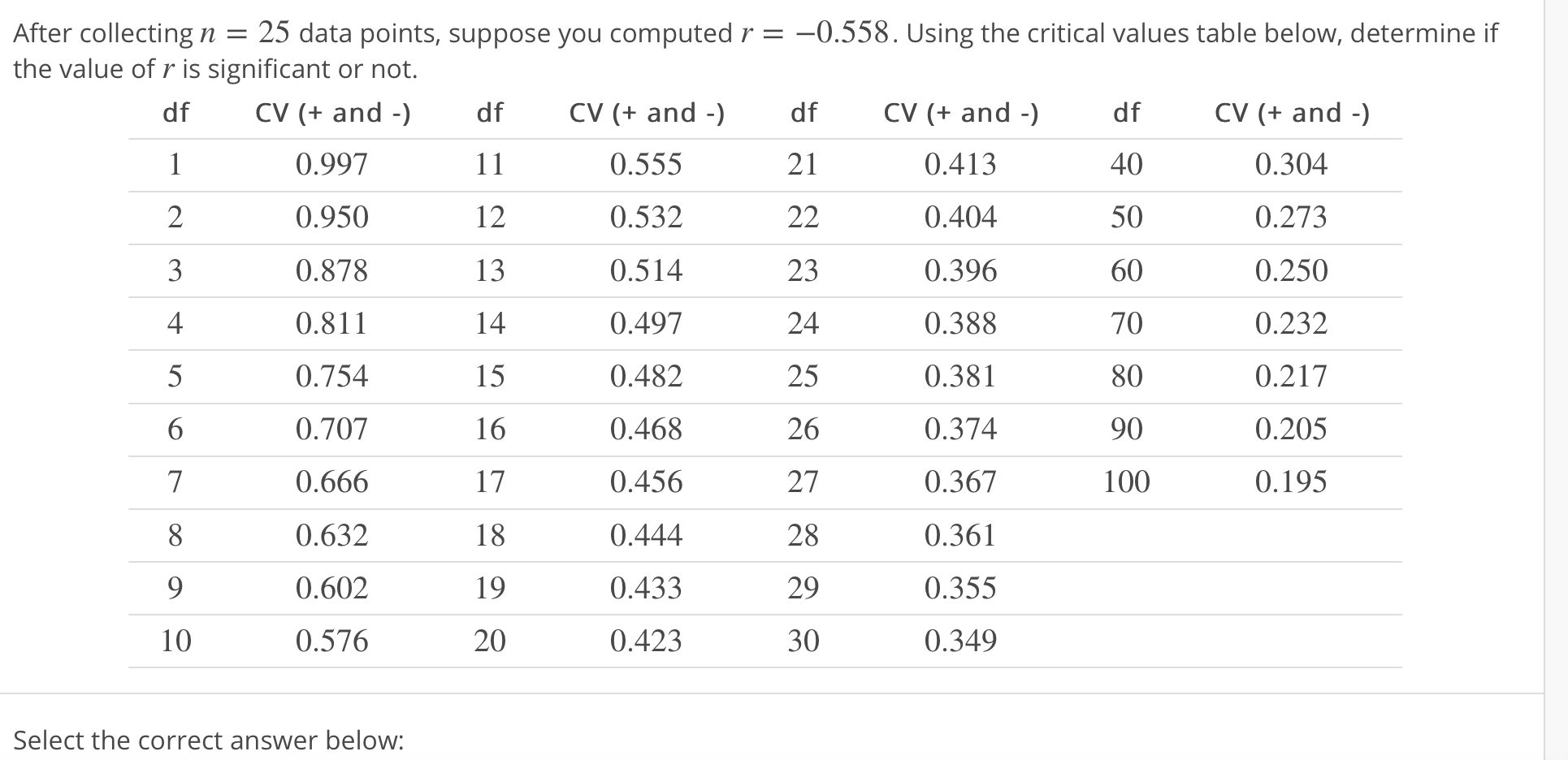After collecting n = 25 data points, suppose you computed rthe value of r is significant or not.-0.558. Using the critical values table below, determine ifdf405060708090100df CV (+ and0.9970.9500.8780.8110.7540.7070.6660.6320.6020.576df CV (+ and - dfCV (+ and -)CV (+ and -)0.3040.2730.2500.2320.2170.2050.195210.5550.5320.5140.4970.4820.4680.4560.4440.4330.4230.4130.4040.3960.3880.3810.3740.3670.3610.3550.3491223413232425262728293015161718192010Select the correct answer below:

Question

Select the correct answer below:

r is significant because it is between the positive and negative critical values.

r is not significant because it is between the positive and negative critical values.

r is significant because it is not between the positive and negative critical values.

r is not significant because it is not between the positive and negative critical values.help_outlineImage TranscriptioncloseAfter collecting n = 25 data points, suppose you computed r the value of r is significant or not. -0.558. Using the critical values table below, determine if df 40 50 60 70 80 90 100 df CV (+ and 0.997 0.950 0.878 0.811 0.754 0.707 0.666 0.632 0.602 0.576 df CV (+ and - dfCV (+ and -) CV (+ and -) 0.304 0.273 0.250 0.232 0.217 0.205 0.195 21 0.555 0.532 0.514 0.497 0.482 0.468 0.456 0.444 0.433 0.423 0.413 0.404 0.396 0.388 0.381 0.374 0.367 0.361 0.355 0.349 12 2 3 4 13 23 24 25 26 27 28 29 30 15 16 17 18 19 20 10 Select the correct answer below: fullscreen
Step 1

From the given information, the sample size n = 25.

Therefore, the degrees of freedom is n – 2 = 25-2 = 23.

The correlation coefficient is -0.558.

From the given table, corresponding to the 23 degrees of freedom, the critical value is ±0.396.

Step 2

Rule for the significant r:

• If the correlation coefficient, r is not between the two critical values that is not between the negative and positive critical values, r is significant.
• Otherwise, r is insignificant.

Here, -0.558<-0.396.

That is, the correlation coefficient is not between the two critical values, -0.396 and 0.396.

Therefore, r is significant.

It can be shown as below,

Step 3

Therefore, the third option is correct that r is significant because it is not between the positive and negative critical values.

1st option is contradictory of 3rd option, hence, 1s option i...

Want to see the full answer?

See Solution

Want to see this answer and more?

Our solutions are written by experts, many with advanced degrees, and available 24/7

See Solution
Tagged in

Statistics